Can you help me with Question #29 in Practice Test 3, Section 4? I found the answer by subtracting multiples of 9 from 122 to find one that was divisible by 5. Is there a better way?

The way I like to go here is to set up equations. If we say left-handed females are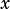and left-handed males are, then we can fill in the table like this:Now we have a system we can solve!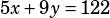We eventually want a number of right-handed females, so let’s solve for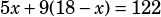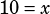Remembering thatis the number of left-handed females, now we just need to multiply by 5 to get the right-handed females: 50.

The last trick here is that the question asks for a probability that a right-handed student being picked at random is female. There are 50 female right-handed students and 122 right-handed students overall, so the probability is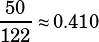.